# PreLab 06

Due by the beginning of lecture on Wednesday, October 8th

In this prelab you will formulate some of the ideas necessary to complete Lab 06. Please type your solutions, and hand in a paper copy before the beginning of class on Wednesday. Remember, no late prelabs allowed!

## Part 0 - Recursive Output

For the following two problems, you'll work out by hand the result of method invocations of the following two recursive methods.

### Function strange

```   def strange(x) :
if x <= 0 :
return 1
else :
return 5 * strange(x-1) - 2
```

### Function weird

```   def weird(x) :
if x > 0 :
print(x,"",end='')
if x%2 == 0 :
weird(x-3)
weird(x-2)
else :
weird(x-1)
```
1) What is the output of print(strange(3))

2) What is the output of weird(8)

## Part 1 - Recursion with Numbers

As we have seen (or will have seen, depending on when you're looking at this), the factorial function can be computed not only using a loop, but via recursion as well. Recall the the typical definition of factorial looks something like this: 0! = 1, 1! = 1, 2! = 2*1 = 2 and 3! = 3*2*1 = 6, and in general, n! = n*(n-1)*...*3*2*1.

But we could also define the factorial function recursively as follows. We'll use fact(n) to denote our recursive representation of n!. We'll define fact(n) = 1 when n is 0 and fact(n) = n*fact(n-1) otherwise. If you think about it, fact(n) gives the exact same values as n!.

3) Give an recursive definition for n^k (n raised to the power of k). That is, you'll probably want to define power(n,k) = ??? when k = 1 and power(n,k) = ??? otherwise. The last component you need to fill in should involve a recursive call to power.

4) Give an recursive definition for the sum of the first n perfect squares. For example, your definition should satisfy sps(1) = 1, sps(2) = 1 + 4 = 5, and sps(3) = 1 + 4 + 9 = 14.

## Part 2 - Recursion with Strings

Here is pseudocode for recursively printing a string s in reverse

```   If the length of s is 0, do nothing
Otherwise, print the last letter of s and reverse s with the last letter removed.
```
5) Give pseudocode for a single function that recursively determines whether a string s is a palindrome (the same forwards as backwards, like "racecar"). You can find the length of s, look up characters in s, and get slices of s, but you should not use any loops.

## Part 3 - Fractal Images

Consider the following sequence of fractal patterns.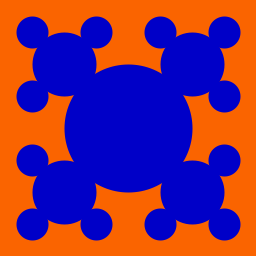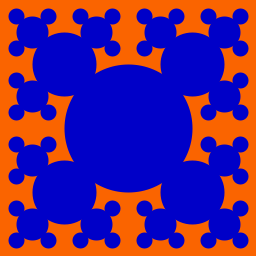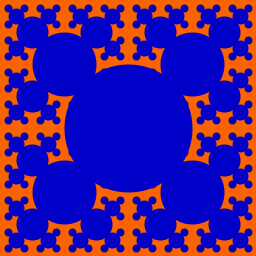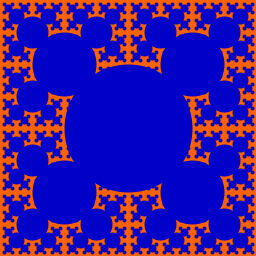Bubbles with recursive depths 1 through 6 respectively.

Each pattern is built entirely out of filled in circles. The pattern can be described recursively as a single filled circle, with four patterns of one lesser depth and half the size, offset to the northwest, northeast, southwest and southeast.

More precisely, at each step in the recursion, you should draw one circle in some quadrant of the canvas, and then divide that quadrant into quarters, each of which will soon contain a new circle, a quarter of the size of the old one.

6) Assume you just drew a circle with radius s//4 in a box of size s, at coordinates (s//2,s//2) and now you want to draw the four smaller circles surrounding it. What will the x and y coordinates of the center of each small circle be?

7) Write pseudo code to recursively draw this fractal pattern. Your function should take a recursive depth, d, representing how deep in the recursion you are, a center x,y, representing the center of the circle, and a size, r, representing the radius of the circle. You should stop drawing when the recursive depth equals 0, and you should subtract one from the depth each time you draw recurse. Your code should have a base case at recursive depth 0, and a recursive case.

Consider the following figure.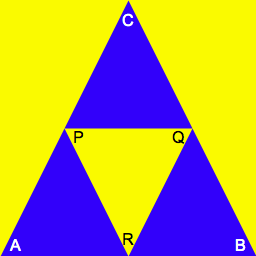Let Ax and Ay denote the x and y coordinates of the corner labelled A in the large triangle above. Let Bx, By, Cx and Cy be definied similarly. Assume P, Q and R each lie at the midpoint of their corresponding edge in the triangle ABC.

8) Specify the x and y coordinates of P, Q and R in terms of A, B and C's x and y coordinates. Don't assume that any coordinates are 0 or that there is any relationship between the points (the triangle ABC pictured happens to be fairly symmetric, but you shouldn't assume this will necessarily be the case).

The gasket pattern, also known as Sierpinski's triangle or Sierpinski's gasket, is built out of trianges. The depth 1 figure includes a single triangle, with lower corners at the lower corners of the pane, and the upper corner centered at the top middle. Each subsequent depth replaces the existing triangle with 3 triangles, each of half the width and half the height as shown below.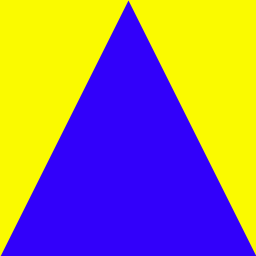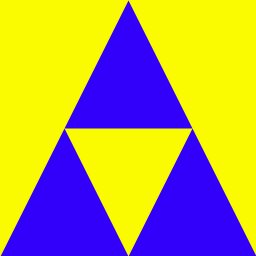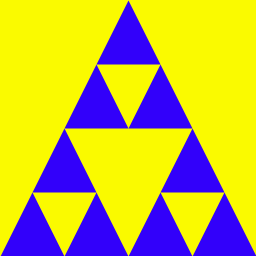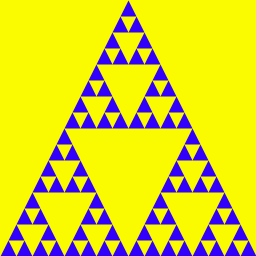Gasket with recursive depths 1 through 6 respectively.

9) There are two distinct recurrences we could use to create this pattern, depending on how we think about the forms. Option 1: draw the carpet (the blue parts). In this case, notice that for a given pattern, all the triangles are of the same size. Thus the creation of triangles only happens as a base case in our recurrence. Option 2: draw the holes (the yellow parts) on top of a single blue triangle. In this case, a recursive call at any depth involves the creation of a triangle (in addition to additional recursive calls, so long as the depth is positive). Pick one of these options, and write pseudocode to implement it.

Consider the fractal patterns below.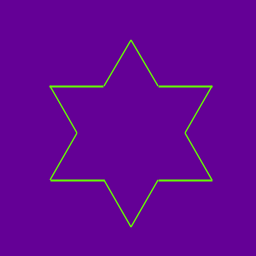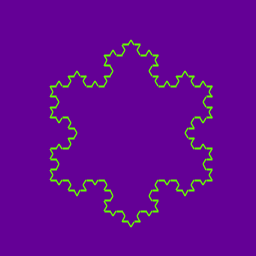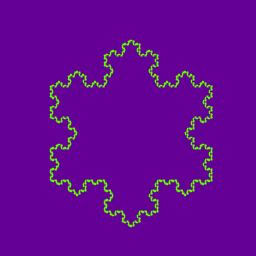Snowflake with recursive depths 1 through 6 respectively.

10) Write code to draw a single side of the snowflake at recursive depth 2. (The first depth more complicated than a triangle.) Use the following drawing methods:
```
rotate(theta)          # rotate pen theta degrees clockwise
drawForward(d)         # draw a length-d line from the current position in the current direction.

```
Assume each segment of the line is some fixed length X. Hint: Every rotation should be some multiple of 60 degrees.

## Honor Code

If you followed the Honor Code in this assignment, write the following sentence attesting to the fact at the top of your homework.

I affirm that I have adhered to the Honor Code in this assignment.